085-1263404

### My Journal

All things Mathematical
18 Oct 2018
##### Question 1

Two pegs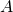and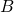are at the same height above the ground, and are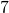feet apart. One end of an inextensible string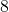feet in length is attached to, and the other end is attached to. A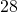lb. weight is suspended from the string at a point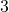feet fromand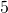feet from. Find the tension in each part of the string.

##### Question 2

Two roads cross at right angles at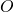. Two men,and, are walking towards.is walking at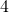m.p.h. on one road,atm.p.h. on the other road. Whenis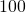yards from,is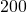yards from. Find the velocity ofrelative to.

Find the distance offromand from, whenandare nearest to each other.

##### Question 3

Prove that the centre of gravity of any triangular lamina is the same as that of three equal masses situated at the vertices of the triangle.is a quadrilateral lamina. A mass equal to one-third the mass of the lamina is placed at the intersection of the diagonals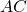and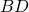. Show that the centre of gravity of the lamina and the mass together is the same as that of four equal masses situated at the vertices of the quadrilateral.

##### Question 4

Two masses oflb. andlb. connected by a light inextensible string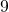feet long, are lying on a smooth horizontal table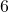feet high. Thelb. mass is at the edge of the table and the other mass isfeet away in a direction perpendicular to the edge. If thelb. mass is pushed gently over the edge of the table, find how long it takes to reach the ground, and how much longer thelb. mass takes to reach the edge of the table.

Find also the change in kinetic energy when thelb. mass is jerked into motion.

##### Question 5

A train ascending an incline ofinaccelerates uniformly from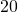m.p.h. to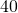m.p.h. in a distance of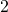miles. Find this acceleration. If the train and the engine together weightons, and if the frictional resistances to motion are equivalent tolb. wt. per ton, find the horse-power at which the engine was working when the speed of the train was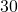m.p.h.

##### Question 6

A point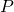is describing a circle, centre, with uniform speed. If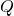is the foot of the perpendicular fromto a fixed diameter, show thatmoves with simple harmonic motion.

Whenisfeet fromits velocity is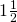feet per second, and when it isfeet fromits velocity isfeet per second towards. Find how longtakes to reachfrom the latter position.

##### Question 7

A gun is fixed at a pointat the top a vertical cliff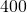feet high.is the foot of the perpendicular fromto the sea. A ship is travelling away from the cliff atfeet per second along a straight line that passes through. When the ship is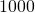feet froma shell is fired from the gun at an angle of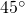and strikes the ship. Find the initial velocity of the shell.

##### Question 8

A mass of one ounce attached to a fixed point by an inextensible stringfeet long, describes a horizontal circle at a uniform rate ofrevolutions per minute. Find the tension in the string and the vertical distance from the fixed point to the plane of the circle.

What vertical distance will the mass rise if its speed is increased to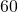revolutions per minute?

##### Question 9

Prove that if a plane surface is immersed in a liquid the total thrust on it due to the liquid is equal to the area of the surface multiplied by the pressure at its center of gravity.

A triangular lamina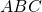is totally immersed in water so that the plane of the lamina makes an angle of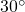with the horizontal plane. The base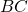isinches in length and the height of the triangle isinches. Find the total thrust of the water of(i) when its vertexis at the surface of the water and its baseis horizontal;

(ii) when its baseis at the surface of the water.

[cubic foot of water weighs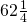lbs.]

##### Citation:

State Examinations Commission (2018). State Examination Commission. Accessed at: https://www.examinations.ie/?l=en&mc=au&sc=ru

Malone, D and Murray, H. (2016). Archive of Maths State Exams Papers. Accessed at: http://archive.maths.nuim.ie/staff/dmalone/StateExamPapers/

##### Licence:

“Contains Irish Public Sector Information licensed under a Creative Commons Attribution 4.0 International (CC BY 4.0) licence”.

The EU Directive 2003/98/EC on the re-use of public sector information, its amendment EU Directive 2013/37/EC, its transposed Irish Statutory Instruments S.I. No. 279/2005, S.I No. 103/2008, and S.I. No. 525/2015, and related Circulars issued by the Department of Finance (Circular 32/05), and Department of Public Expenditure and Reform (Circular 16/15 and Circular 12/16).

Note. Circular 12/2016: Licence for Re-Use of Public Sector Information adopts CC-BY as the standard PSI licence, and notes that the open standard licence identified in this Circular supersedes PSI General Licence No: 2005/08/01.